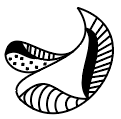Question 1

# Which of the option figures when rotated $$180^{\circ}$$ clockwise and then $$45^{\circ}$$ anticlockwise will result in the given question figure?• Free SSC Study Material - 18000 Questions
• 230+ SSC previous papers with solutions PDF